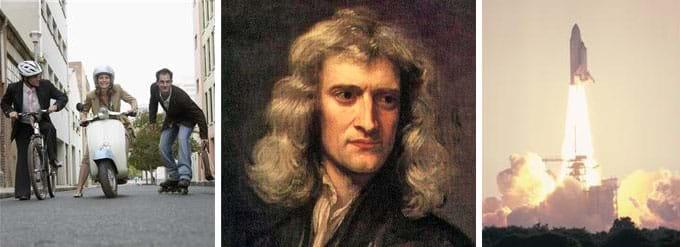# UnitWhat Are Newton's Laws?

(0 Ratings)

### Quick Look

Choose From: 3 lessons and 1 activities

Subject Areas: Physical Science, PhysicsNewton's laws of motion explain the movement of everyday objects and are important to engineers who design machines, structures and products.copyrightCopyright © (left, right) 2004 Microsoft Corporation, One Microsoft Way, Redmond, WA 98052-6399 USA. All rights reserved; (middle) 1689 Sir Godfrey Kneller, Wikimedia Commons http://office.microsoft.com/en-us/images/results.aspx?qu=scooter&ex=1#ai:MP900425518| http://commons.wikimedia.org/wiki/File:GodfreyKneller-IsaacNewton-1689.jpg http://office.microsoft.com/en-us/images/results.aspx?qu=vehicle&ex=1#ai:MP900414117|mt:2|

### Summary

Through a series of three lessons and one activity, students are introduced to inertia, forces and Newton's three laws of motion. For each lesson, a combination of class demonstrations and PowerPoint® presentations are used to explain, show and relate the concepts to engineering. Lesson 1 starts with inertia, forces and Newton's first law of motion. Lesson 2 builds on lesson 1 with a review and then introduces Newton's second law of motion. Lesson 3 builds on the previous two lessons with a review and then introduces Newton's third law of motion. In a culminating activity, students apply their knowledge of forces, friction, acceleration and gravity in an experiment to measure the average acceleration of a textbook pulled along a table by varying weights, and then test the effects of friction on different surfaces.
This engineering curriculum aligns to Next Generation Science Standards (NGSS).

### Engineering Connection

Engineers apply basic physics concepts such as Newton's laws of motion in a wide range of situations such as designing all sorts of stationary and moving objects, from the massive to the delicate. This includes structures, vehicles and objects such as bridges, rockets, aircraft, seat belts, door knobs and medicine delivery systems. To design objects that perform as we want and are safe, engineers must fully understand the workings of the natural physical laws. Learning how Newton's laws apply in everyday situations and devices enables students to be able to describe how objects move and prepares them for the study of more complex physics concepts.

### Unit Overview

Newton's first law of motion: Unless an unbalanced force acts on an object, an object at rest stays at rest and an object in motion stays in motion.

Newton's second law of motion: Force = mass x acceleration (aka F=ma)

Newton's third law of motion: For every action, there is an equal and opposite reaction.

### Educational Standards Each TeachEngineering lesson or activity is correlated to one or more K-12 science, technology, engineering or math (STEM) educational standards. All 100,000+ K-12 STEM standards covered in TeachEngineering are collected, maintained and packaged by the Achievement Standards Network (ASN), a project of D2L (www.achievementstandards.org). In the ASN, standards are hierarchically structured: first by source; e.g., by state; within source by type; e.g., science or mathematics; within type by subtype, then by grade, etc.

See individual lessons and activities for standards alignment.

### Unit Schedule

Each lesson and activity is designed to take 60-minutes, for a total of four 60-minute sessions for the unit. The teaching style, depth of instruction and student level may cause the lessons to take more or less time.

The suggested order to conduct the lessons and activity in the unit:

Day 4: Sliding Textbooks

### More Curriculum Like This

Upper Elementary LessonWhat Is Newton's First Law?

Students are introduced to the concepts of force, inertia and Newton's first law of motion: objects at rest stay at rest and objects in motion stay in motion unless acted upon by an unbalanced force. Students learn the difference between speed, velocity and acceleration, and come to see that the cha...

High School LessonA Tale of Friction

High school students learn how engineers mathematically design roller coaster paths using the approach that a curved path can be approximated by a sequence of many short inclines. They apply basic calculus and the work-energy theorem for non-conservative forces to quantify the friction along a curve...

Upper Elementary LessonNewton Gets Me Moving

Students explore motion, rockets and rocket motion while assisting Spacewoman Tess, Spaceman Rohan and Maya in their explorations. First they learn some basic facts about vehicles, rockets and why we use them. Then, they discover that the motion of all objects—including the flight of a rocket and mo...

Upper Elementary LessonWhat Is Newton's Third Law?

Students are introduced to Newton's third law of motion: For every action, there is an equal and opposite reaction. They practice identifying action-reaction force pairs for a variety of real-world examples, and draw and explain simplified free-body diagram vectors (arrows) of force, velocity and ac...

### Assessment

The individual lessons include assessment suggestions to implement throughout the unit (discussion questions, exit ticket, homework, etc.). In addition, a summative assessment unit quiz is provided as an attachment to lesson 3, to be administered after completion of all three lessons and the activity.

### Other Related Information

The San Francisco Exploratorium provides a list of 17 classroom demonstrations of Newton's laws of motion that you may want to incorporate into the unit, in addition to those already provided. See http://www.exo.net/~donr/activities/Newton's_Laws_Demonstrations.pdf

### Contributors

Elizabeth Anthony, Scott Strobel, Jacob Teter

### Supporting Program

RESOURCE GK-12 Program, College of Engineering, University of California Davis

### Acknowledgements

The contents of this digital library curriculum were developed by the Renewable Energy Systems Opportunity for Unified Research Collaboration and Education (RESOURCE) project in the College of Engineering under National Science Foundation GK-12 grant no. DGE 0948021. However, these contents do not necessarily represent the policies of the National Science Foundation, and you should not assume endorsement by the federal government.